# Parallel Circuit

## Key Stage 3

### Meaning

A Parallel Circuit is an electrical circuit with two or more paths the current can flow along.

In a parallel circuit the current is split at junctions before taking a different path.
Switches can be placed in a Parallel Circuit to allow current along one path at a time.
Components placed in parallel with each other have the same Potential Difference across them.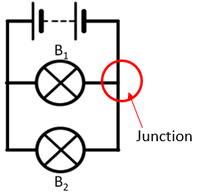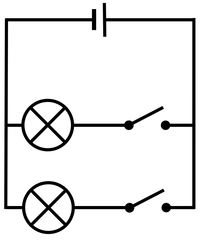The current from the battery splits at the junction sharing the current between the two bulbs. The two bulbs in this parallel circuit can be switched on and off separately.

### Examples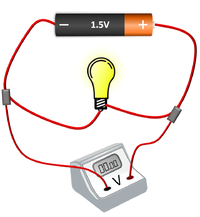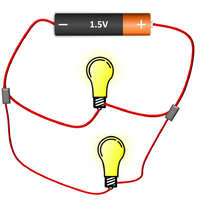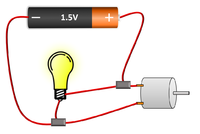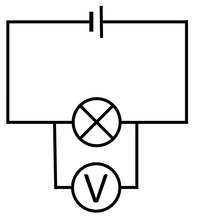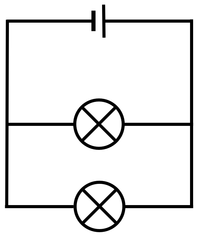The cell, bulb and voltmeter are in parallel so they have the same potential difference across them. The two bulbs are in parallel so they have the same potential difference across them but may have a different current passing through them. The bulb and motor are in parallel so they have the same potential difference across them but may have a different current passing through them.

## Key Stage 4

### Meaning

A Parallel Circuit is an electrical circuit with two or more paths the current can flow along.

In a parallel circuit the current is split at junctions before taking a different path.
Switches can be placed in a parallel circuit to allow current along one path at a time.
Components placed in parallel with each other have the same potential difference across them.The current from the battery splits at the junction sharing the current between the two bulbs. The two bulbs in this parallel circuit can be switched on and off separately.

### ExamplesThe cell, bulb and voltmeter are in parallel so they have the same potential difference across them. The two bulbs are in parallel so they have the same potential difference across them but may have a different current passing through them. The bulb and motor are in parallel so they have the same potential difference across them but may have a different current passing through them.

### Resistors in Parallel

NB: You only need to know what happens with identical resistors in parallel.

When identical resistors are added in parallel there are more paths for the electricity so the resistance is reduced.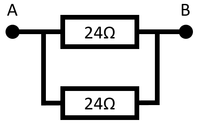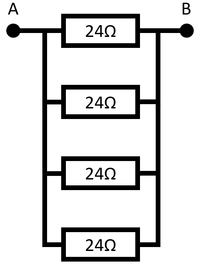Two identical resistors in parallel gives twice the number of paths, so has half the resistance. The resistance between points A and B is 12Ω. Three identical resistors in parallel gives three times the number of paths, so has a third of the resistance. The resistance between points A and B is 8Ω. Four identical resistors in parallel gives four times the number of paths, so has a quarter of the resistance. The resistance between points A and B is 6Ω.